Multiplying Rational Expressions (page 1 of 2)With regular fractions, multiplying and dividing is fairly simple, and is much easier than adding and subtracting. The situation is much the same with rational expressions (that is, with polynomial fractions). The only major problem I have seen students having with multiplying and dividing rationals is with illegitimate cancelling, where they try to cancel terms instead of factors, so I'll be making a big deal about that as we go along.

Remember how you multiply regular fractions: You multiply across the top and bottom. For instance: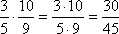And you need to simplify, whenever possible: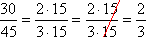While the above simplification is perfectly valid, it is generally simpler to cancel first and then do the multiplication, since you'll be dealing with smaller numbers that way. In the above example, the 3 in the numerator of the first fraction duplicates a factor of 3 in the denominator of the second fraction, and the 5 in the denominator of the first fraction duplicates a factor of 5 in the numerator of the second fraction. Since anything divided by itself is just 1, we can "cancel out" these common factors (that is, we can ignore these forms of 1) to find a simpler form of the fraction: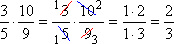This process (cancelling first, then multiplying) works with rational expressions, too.

• Simplify the following expression:
•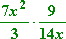Simplify by cancelling off duplicate factors: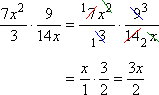(If you're not sure how the variable parts were simplified above, you may want to review how to simplify expressions with exponents.)

Then the answer is:

Advertisement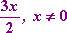Why did I add the "for x not equal to 0" notation after the simplified fraction? Because the original expression was not defined at x = 0 (since this would have caused division by zero in the second of the two original fractions). For the two expressions, the original one and the simplified one, to be "equal" in technical terms, their domains have to be the same; they have to be defined for the same x-values. Since the simplified fraction, 3x/2, has no division-by-zero problem at x = 0, it is not, strictly-speaking, "equal" to the original expression. To make the simplified form truly equal to the original form, I have to explicitly state this "x cannot be zero" exclusion.

Warning: Your particular textbook or instructor might not make this distinction. If you're not sure if your teacher cares about this technicality, please make sure to ask before the next test.

• Multiply and simplify the following:
•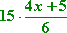Many students find it helpful to convert the "15" into a fraction. This can make the factors a little more obvious, so one can see more clearly what can cancel with what.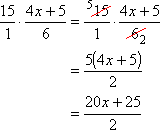Can I cancel off the 2 into the 20? No! When I have a fraction like this, there are understood parentheses around any sums of terms, like this: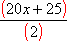I can only cancel off factors (the entire contents of a set of parentheses); I can NOT cancel terms (part of what is inside a set of parentheses). Barging inside those parentheses, willy-nilly hacking x's and y's and arms and legs off the poor polynomial, doesn't "simplify" anything; it just leaves the sad little polynomial lying there on the floor, quivering and bleeding and oozing and whimpering...

Okay, maybe not; but you get the point: Never reach inside the parentheses and hack off part of the contents. Either you cancel off the entire contents of a parenthetical or factor with a matching parenthetical or factor from the other side of the fraction line, or you do NOT cancel anything at all.

The only thing that I can factor out of the 20x + 25 in the numerator is a 5, and that factor doesn't cancel off with the 2 underneath, so, for this rational, there is no further reduction to be done. Then my final answer is: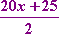Copyright © Elizabeth Stapel 2003-2011 All Rights Reserved

• Multiply and simplify the following expression:
•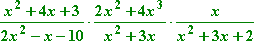Some students, when faced with this problem, will do something like this: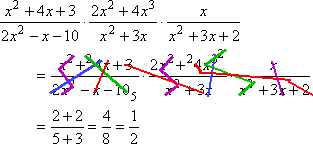Can they really "cancel" like this? (Think "bleeding"...) Is this even vaguely legitimate? (Oozing...) Has this student done anything at all correctly? (Flopping, whimpering...) No, no, and no!

You can not cancel terms;
you can only cancel factors.

Since I can only cancel factors, my first step in this simplification has to be to factor all the numerators and denominators. Once I've factored everything, I can cancel off any factor that is mirrored on the two sides of the fraction line. The legitimate simplification looks like this: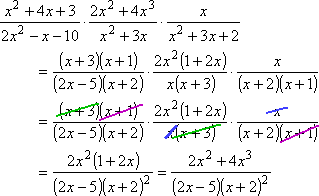Can I now cancel off some 2's? (Tears are welling up in the polynomial's eyes...) Can I cancel off any of the x's with the x2? (Now the polynomial is starting to cry...) Hmm??? No! The x's are only part of their respective factors; they are not stand-alone factors, so they can't cancel off with anything.

Then my answer, taking note of the trouble-spots (the division-by-zero problems) that I removed when I cancelled the common factors, is: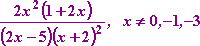The "x not equal to 0, –1 or –3" came from the factors that I cancelled off; your book may not require this information as part of your answer.

Note: For reasons which will become clear when you are adding rational expressions, it is customary to leave the denominator factored, as shown above. At this stage, your book may or may not want the numerator factored. You should recognize, in any case, that "(2x – 5)(x + 2)2" is the same thing as "2x3 + 3x2 – 12x – 20", and get in the habit of converting the form of your answer if your book or instructor expects it written in a particular way.

Top  |  1 | 2  |  Return to Index  Next >>

 Cite this article as: Stapel, Elizabeth. "Multiplying Rational Expressions." Purplemath. Available from     https://www.purplemath.com/modules/rtnlmult.htm. Accessed [Date] [Month] 2016

MathHelp.com Courses
 Copyright © 2021  Elizabeth Stapel   |   About   |   Terms of Use   |   Linking   |   Site Licensing Contact Us